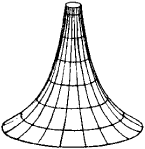# Pseudosphere

Also found in: Dictionary, Wikipedia.

## pseudosphere

[′süd·ə‚sfir]
(mathematics)
The pseudospherical surface generated by revolving a tractrix about its asymptote.

## Pseudosphere

the surface of constant negative curvature formed by the rotation of a tractrix about its asymptote (see Figure 1). Its name emphasizes both its similarity to and difference from a sphere, which is an example of a surface with constant positive curvature. The pseudosphere is of particular interest because figures drawn on smooth parts of this surfaceobey the laws of Lobachevskian non-Euclidean geometry. This fact, established in 1868 by E. Beltrami, was of great importance in the dispute over the reality of Lobachevskian geometry.

References in periodicals archive ?
Then, by using a perturbation method, we were able to analytically calculate the shape of the buckled membrane and show that it is a pseudosphere.
1998] give a simple round-by-round construction that unifies the synchronous, semi-synchronous, and asynchronous message-passing models of distributed computation within a common formalism based on a topological construction called a pseudosphere.
He simply cut a series of "latitude" and "longitude" circles out of plywood and built a pseudosphere 231/2 inches in diameter.

Site: Follow: Share:
Open / Close